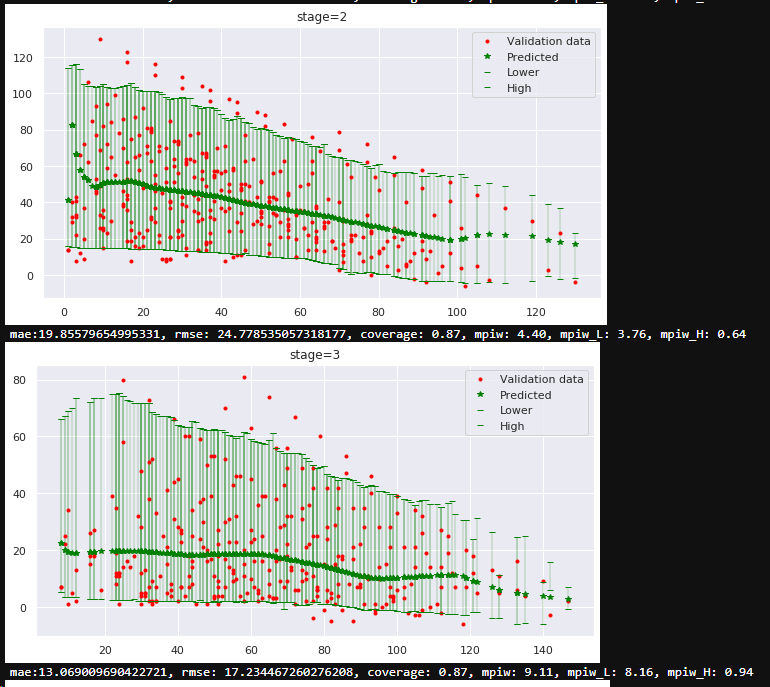# learn prediction intervals (variance, noise)

The problem: I'm looking to emit prediction intervals for each predicted value (the mean) in regression. I need that these intervals cover say 90% of true values and be as narrow as possible. In other words I want to learn and emit variance (or noise) which can't be explained by features of the model in each region of data - each sample would have different intervals determined by input vector. For example: Predicting income by number of education years. Given there is no additional data we have I would expect lower variance of income for lower education and higher variance for higher education. Another example - predicting how much years left to leave, by age and health data of a person. Young would have larger variance, while old lower, and old and unhealthy even more lower.

There are two main methods I'm aware of to do it: - non-parametric methods - using Quantile Loss, where trying to get 90% of coverage we can train three models: Quantile:alpha=0.5 for the median and alpha=0.1 and 0.9 for the lower and higher bounds (quantiles) respectfully. - parametric method - suppose some distribution of the noise, and learn it's parameters. For example to get 90% coverage suppose Normal distribution and train models for mean and stdev. Then calculate the interval using stdev. - bayesian methods, essentially parametric as well, but the modeling is done using probabilistic methods.

The question: As mentioned I want the interval be as narrow as possible but still satisfy the needed coverage, which means learning separate models for quantiles or parameters for parametric methods wouldn't provide optimal solution in terms of coverage and width. I'm looking for the loss function which can optimize two things simultaneously. Is there something builtin already in some library and if not what would be the simplest way to implement it? Both parametric and non parametric methods are accepted. Thanks in advance! Alexander

Example The data in example is simulated: 2 independent variables - stage (categorical) and age (axis X), axis Y is the predicted value. The bounds and mean created by 3 separate quantile models. But real data is much more complex, so separate models approach not creating nice results.• Note that $\alpha=0.5$ will give you the median, which will be different from the mean if your distribution is not symmetrical. Jan 16, 2020 at 10:24
• thanks, you completely right, I meant for mean in terms of loss optimization but things mixed me up. Fixed in question. Jan 16, 2020 at 12:10